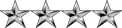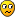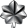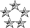## Need help on Permutations

Mathematics for Computing
Nipuna
ModeratorPosts: 2729
Joined: Mon Jan 04, 2010 8:02 pm
Location: Deraniyagala,SRI LANKA

### Need help on Permutations

Hello New Problem.

I can Solve questions like these.

Q= You have given 5 different letters k, l, m, n, o
What is the number of permutations that you can have by taking 3 letters at a time ?

P(n,r)=n!/(n-r)!
=5!/(5-3)!
=5!/2!
=5.4.3.2.1/2.1
=120/2

=60

And can Resolve Combination Questions too.

But Don't understand How to do ones like these?What is the number of permutations that you can have by taking 3 letters at a time and the first letter to be m or n?
Saman
Lieutenant ColonelPosts: 828
Joined: Fri Jul 31, 2009 10:32 pm
Location: Mount Lavinia

### Re: Need help on Permutations

Again Permutations & Combinations (One of the A/L Maths topics and also done in uni level maths). This subject is very easy.

Here are the Wiki articles on this subject.
Permutation
Combination

See following video tutorials under Discrete Mathematical Structures Tutorial

Lecture-27 - Permutations and Combinations
Lecture 28 - Permutations and combinations
Lecture 29 - Permutations and Combinations (Contd...)
NeoPosts: 2642
Joined: Wed Jul 15, 2009 2:07 am
Location: Colombo

### Re: Need help on Permutations

For the guy who don't have an idea on the subject see my little tutorial. What is Permutations & Combinations?
But Don't understand How to do ones like these?What is the number of permutations that you can have by taking 3 letters at a time and the first letter to be m or n?
Very easy when you get it right. Just notice the 2nd tree of your first post.
We had 3 letters there {a, b,c}. We were asked to get the permutations and we had 6.
Note the first letter on them. We had 2 'a's, 2 'b's and 2 'c's as the first letter.
So to get the number of permutations which starts with letter 'a', you need to divide the result by n (n=3).
So if you were asked to get the number of permutations that starts with letter a or b. It would be,

(3P3 / 3) * 2 = (6 / 3) * 2 = 4

Our set S is {k, l, m, n, o}. n= 5. We were asked to pick 3 letters at a time means k = 3.
5P3 = 5! / (5 - 3)! = 5! / 2! = 5 x 4 x 3 = 60
On these 60 permutations, we have equal number of 'k', 'l', 'm', 'n' and 'o' letters.
Number of permutations from 1 letter is, 60 / 5 = 12.
So, Number of permutations from 2 letters is, (60 / 5) x 2 = 24

I'll make the question a bit difficult nowLet's say we were given the same set {k, l, m, n, o}. Can you tell me the number of permutations that you can get when you chose 3 letters with first letter 'm' and the second letter not 'm'. Just evaluate your answer and see my answer.

5P3 = 60
To have first letter m = 60 / 5 = 12
When you select m once, you can't select it again. So the answer is obviously 12.

Okay another easy one.
From the same set {k, l, m, n, o}, you were asked to pick 3 numbers where the first letter to be 'k' and the second to be 'n'.
5P3 = 60
First letter to be 'k' = 60 / 5 = 12
Out of these 12, the second letter also repeats equal times. Since we have already selected the first letter, we only have 4 letters now. So each letter repeats 12 / 4 times. So the answer is 3.

See how easy the permutations are. You just need to read the question carefully. That's all. Combinations are even more easier.
Nipuna
ModeratorPosts: 2729
Joined: Mon Jan 04, 2010 8:02 pm
Location: Deraniyagala,SRI LANKA

### Re: Need help on Permutations

Cool. And Thanks My Dear TeacherI will read later Since I have to go now.

Thanks Again.
Nipuna
ModeratorPosts: 2729
Joined: Mon Jan 04, 2010 8:02 pm
Location: Deraniyagala,SRI LANKA

### Re: Need help on Permutations

Cool. I got ItThis is a Trick Questions
I'll make the question a bit difficult nowLet's say we were given the same set {k, l, m, n, o}. Can you tell me the number of permutations that you can get when you chose 3 letters with first letter 'm' and the second letter not 'm'. Just evaluate your answer and see my answer.
After reading It well. It's Easy. It's same as Which is " How much permutations we Get that Beginning with "m""

Thanks a Lot. And I have Questions with that Nasty Pigeon hole Principal and Pascal's Triangle I will ask them Later.

I get what is Pigeonhole Principal. It says "If there are n holes and we have n+1 pigeon, Then for a one hole there are more than one Pigeon " That can even Understand by a 5 Year Kid. But the Questions I can see on papers, I don't get them.

Here are They Pigeonhole Principal Thread
Binomial Theorem And Binomial Coefficients
Pascal's Principal